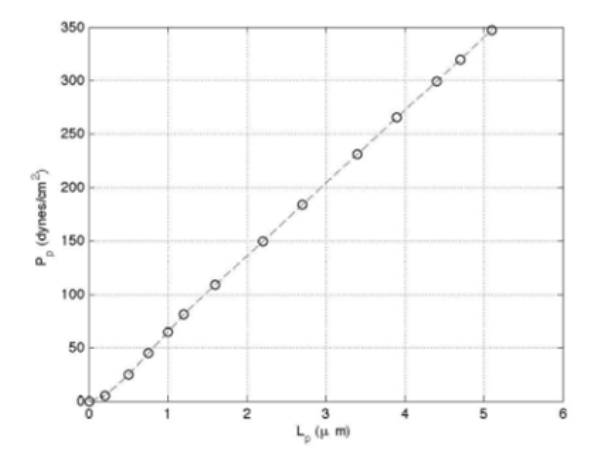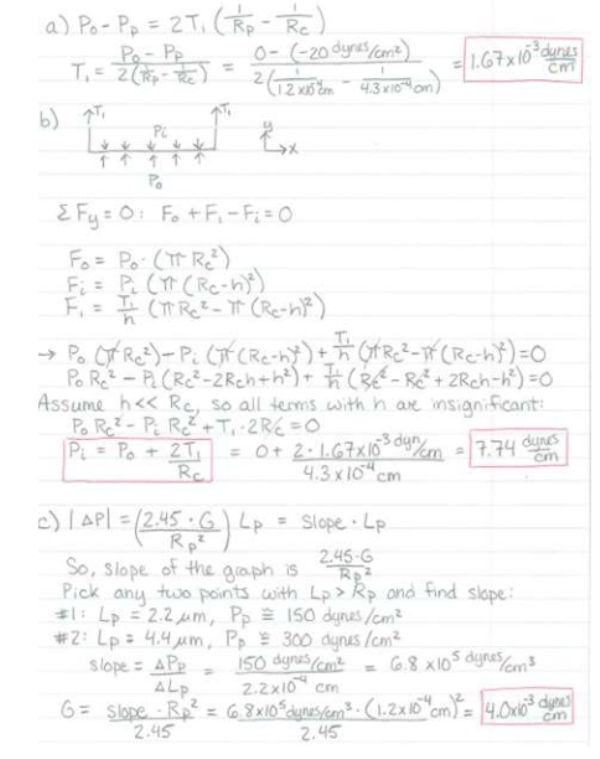For the RBC micropipet experiment below, a micropipet pressure Pp of -20 dynes/cm^2 is applied. The pipet radius Rp is 1.2 um, the maximum cell radius Rc is 4.3 um, Lp is 0.9 um, and P0 is 0 dynes/cm^2. Find the membrane tension component T at the interface of the micropipet and cell. b) We solved for the membrane tension component T in part a, Now, assume that T is the same at the bottom of the cell and that the cell can be represented as a simple hockey puck disc shape. A free body diagram of the bottom of the disk is shown below, where Rc is the cell radius (4.3 um). h is the membrane thickness, and P0 is the external pressure. Perform a force balance to generate an equation for Pi and calculate Pi. c) Like most biological material, RBC exhibit characteristics of both solid and fluid materials. During aspiration, RBC membranes can resist aspiration due to their shear elasticity when Lp is greater than Rp.For the RBC micropipet experiment below, a micropipet pressure Pp of -20 dynes/cm^2 is applied. The pipet radius Rp is 1.2 um, the maximum cell radius Rc is 4.3 um, Lp is 0.9 um, and P0 is 0 dynes/cm^2. Find the membrane tension component T at the interface of the micropipet and cell. b) We solved for the membrane tension component T in part a, Now, assume that T is the same at the bottom of the cell and that the cell can be represented as a simple hockey puck disc shape. A free body diagram of the bottom of the disk is shown below, where Rc is the cell radius (4.3 um). h is the membrane thickness, and P0 is the external pressure. Perform a force balance to generate an equation for Pi and calculate Pi. c) Like most biological material, RBC exhibit characteristics of both solid and fluid materials. During aspiration, RBC membranes can resist aspiration due to their shear elasticity when Lp is greater than Rp.

biomechanics page 1 biomechanics biomechanics biomechanics biomechanics biomechanics biomechanics biomechanics biomechanics page 2